Chapter Three STOICHIOMETRY Chemical Stoichiometry Stoichiometry The study

• Slides: 40Chapter Three: STOICHIOMETRY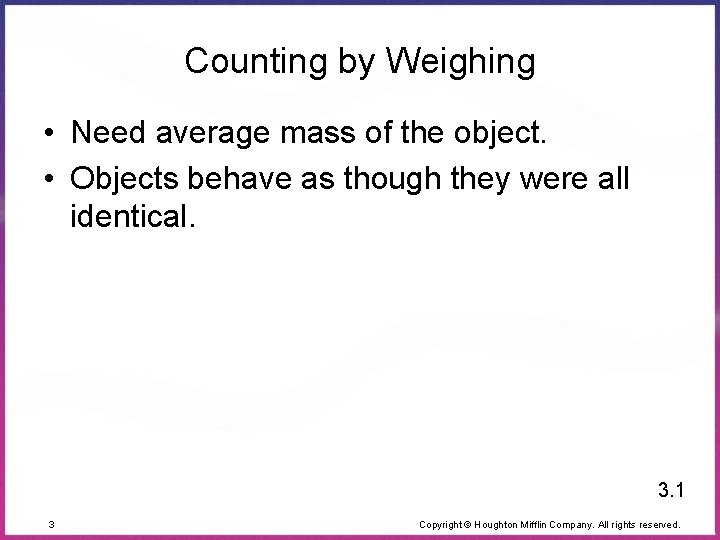Counting by Weighing • Need average mass of the object. • Objects behave as though they were all identical. 3. 1 3 Copyright © Houghton Mifflin Company. All rights reserved.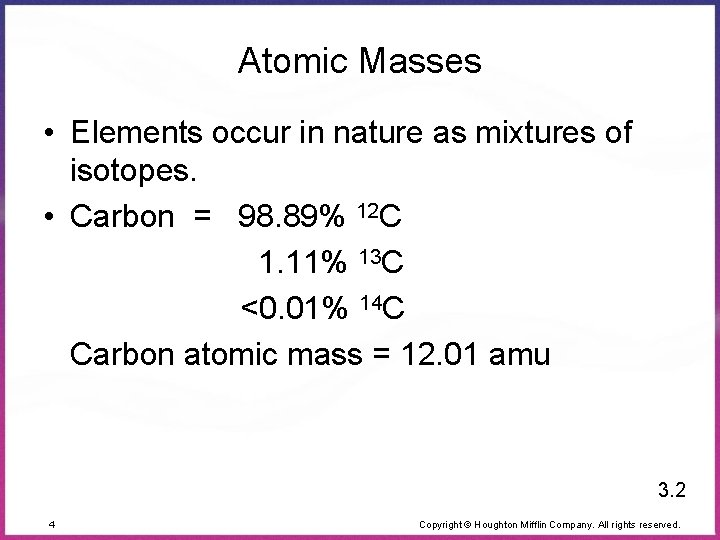Atomic Masses • Elements occur in nature as mixtures of isotopes. • Carbon = 98. 89% 12 C 1. 11% 13 C <0. 01% 14 C Carbon atomic mass = 12. 01 amu 3. 2 4 Copyright © Houghton Mifflin Company. All rights reserved.The Mole • The number equal to the number of carbon atoms in exactly 12 grams of pure 12 C • 1 mole of anything = 6. 022 x 1023 units of that thing (Avogadro’s number) • 1 mole C = 6. 022 x 1023 C atoms = 12. 01 g C 3. 3 6 Copyright © Houghton Mifflin Company. All rights reserved.Molar Mass • Mass in grams of one mole of the substance: Molar Mass of N = 14. 01 g/mol Molar Mass of H 2 O = 18. 02 g/mol (2 × 1. 008) + 16. 00 Molar Mass of Ba(NO 3)2 = 261. 35 g/mol 137. 33 + (2 × 14. 01) + (6 × 16. 00) 3. 4 7 Copyright © Houghton Mifflin Company. All rights reserved.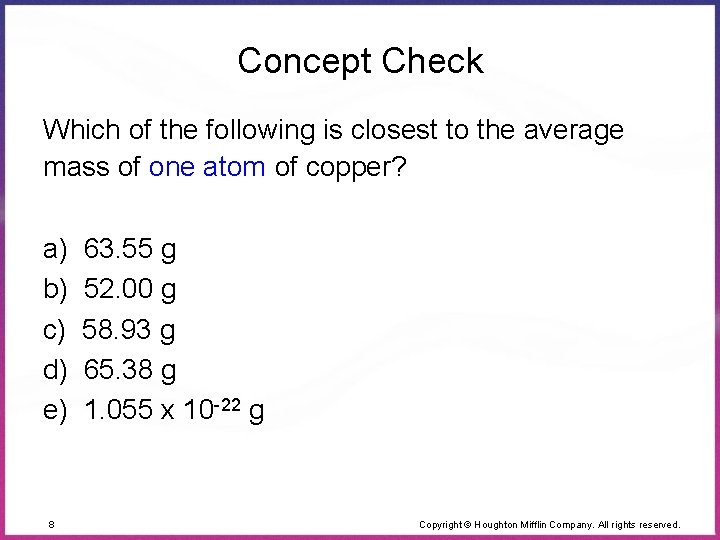Concept Check Which of the following is closest to the average mass of one atom of copper? a) 63. 55 g b) 52. 00 g c) 58. 93 g d) 65. 38 g e) 1. 055 x 10 -22 g 8 Copyright © Houghton Mifflin Company. All rights reserved.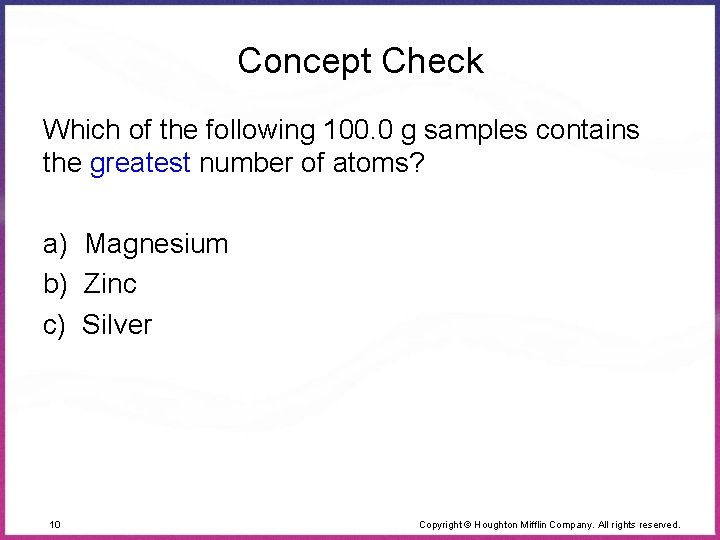Concept Check Which of the following 100. 0 g samples contains the greatest number of atoms? a) Magnesium b) Zinc c) Silver 10 Copyright © Houghton Mifflin Company. All rights reserved.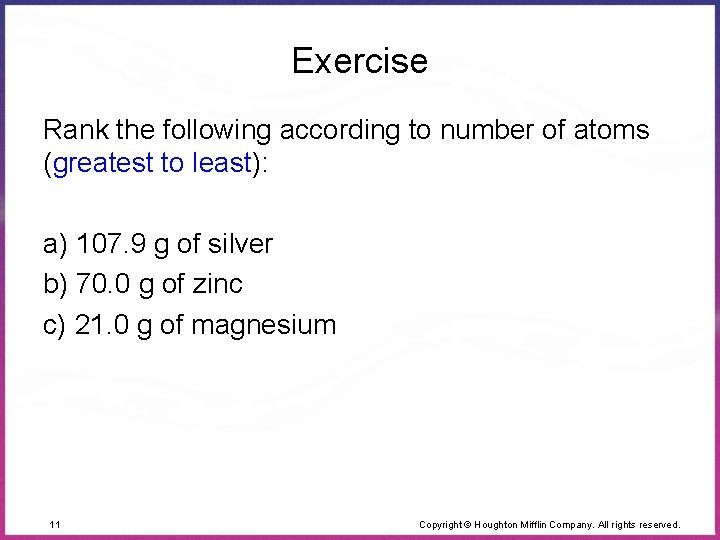Exercise Rank the following according to number of atoms (greatest to least): a) 107. 9 g of silver b) 70. 0 g of zinc c) 21. 0 g of magnesium 11 Copyright © Houghton Mifflin Company. All rights reserved.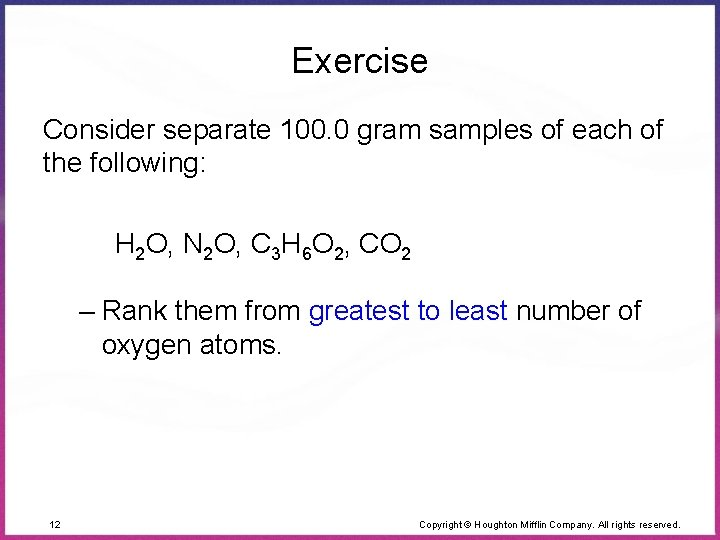Exercise Consider separate 100. 0 gram samples of each of the following: H 2 O, N 2 O, C 3 H 6 O 2, CO 2 – Rank them from greatest to least number of oxygen atoms. 12 Copyright © Houghton Mifflin Company. All rights reserved.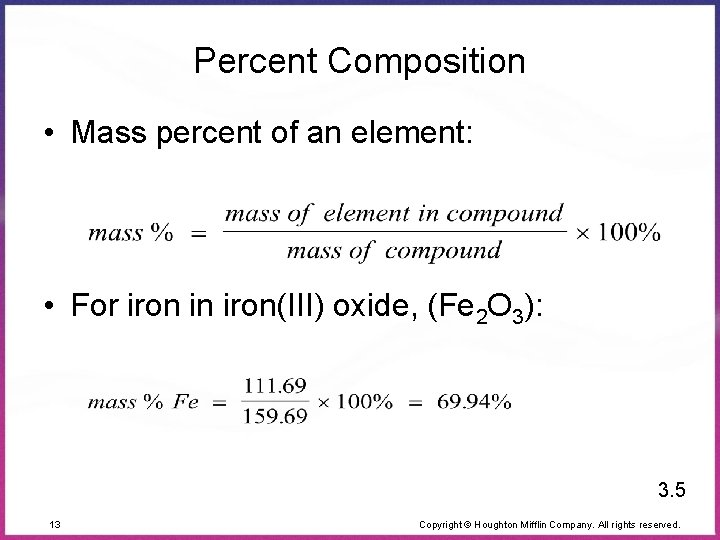Percent Composition • Mass percent of an element: • For iron in iron(III) oxide, (Fe 2 O 3): 3. 5 13 Copyright © Houghton Mifflin Company. All rights reserved.Exercise Consider separate 100. 0 gram samples of each of the following: H 2 O, N 2 O, C 3 H 6 O 2, CO 2 – Rank them from highest to lowest percent oxygen by mass. 14 Copyright © Houghton Mifflin Company. All rights reserved.Formulas • Molecular formula = (empirical formula)n [n = integer] • Molecular formula = C 6 H 6 = (CH)6 – Actual formula of the compound • Empirical formula = CH – Simplest whole-number ratio 3. 6 15 Copyright © Houghton Mifflin Company. All rights reserved.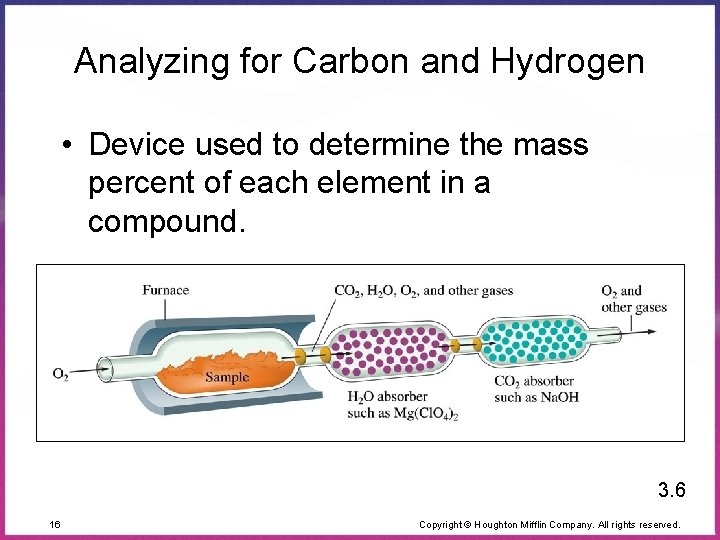Analyzing for Carbon and Hydrogen • Device used to determine the mass percent of each element in a compound. 3. 6 16 Copyright © Houghton Mifflin Company. All rights reserved.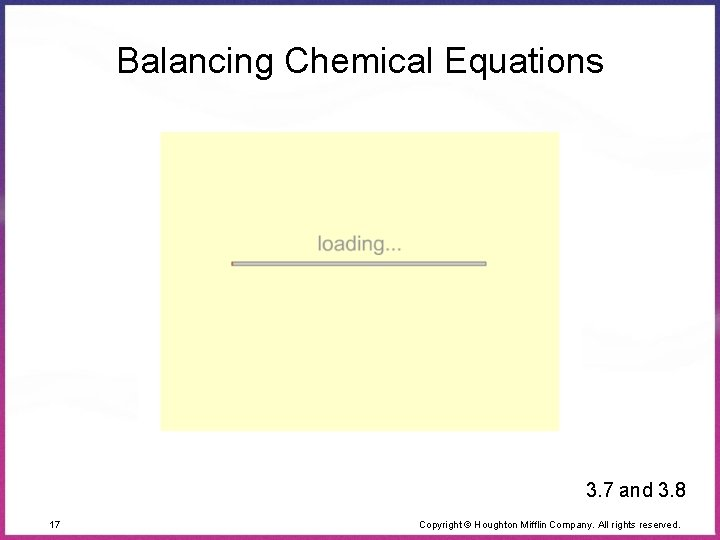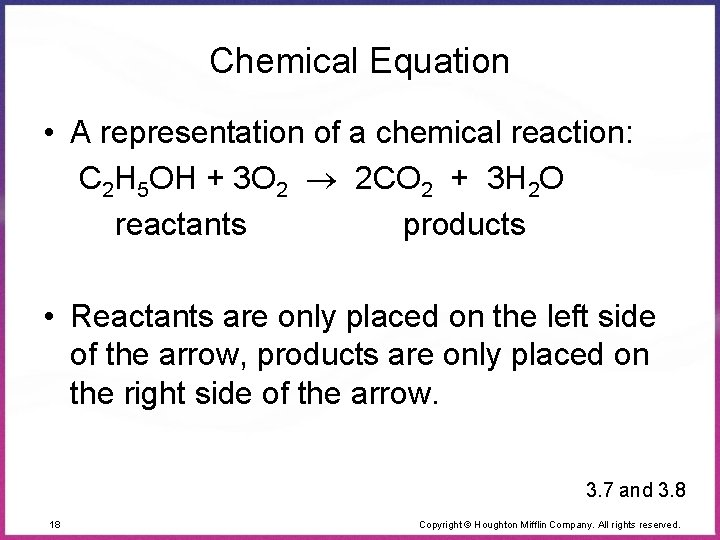Chemical Equation • A representation of a chemical reaction: C 2 H 5 OH + 3 O 2 2 CO 2 + 3 H 2 O reactants products • Reactants are only placed on the left side of the arrow, products are only placed on the right side of the arrow. 3. 7 and 3. 8 18 Copyright © Houghton Mifflin Company. All rights reserved.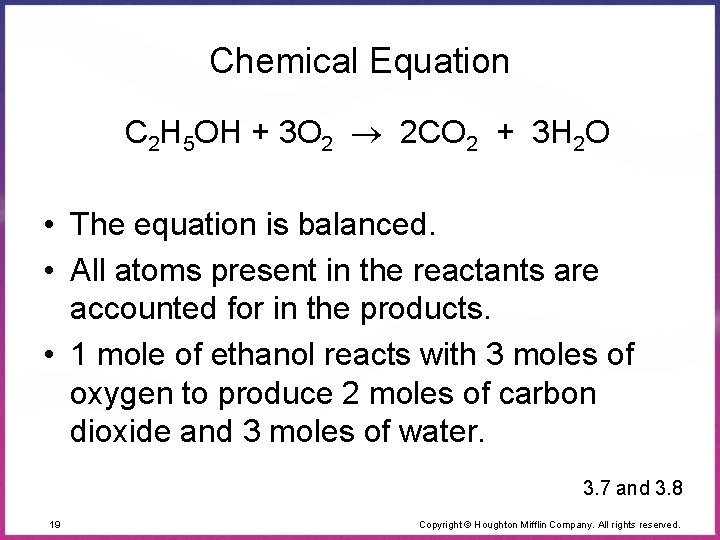Chemical Equation C 2 H 5 OH + 3 O 2 2 CO 2 + 3 H 2 O • The equation is balanced. • All atoms present in the reactants are accounted for in the products. • 1 mole of ethanol reacts with 3 moles of oxygen to produce 2 moles of carbon dioxide and 3 moles of water. 3. 7 and 3. 8 19 Copyright © Houghton Mifflin Company. All rights reserved.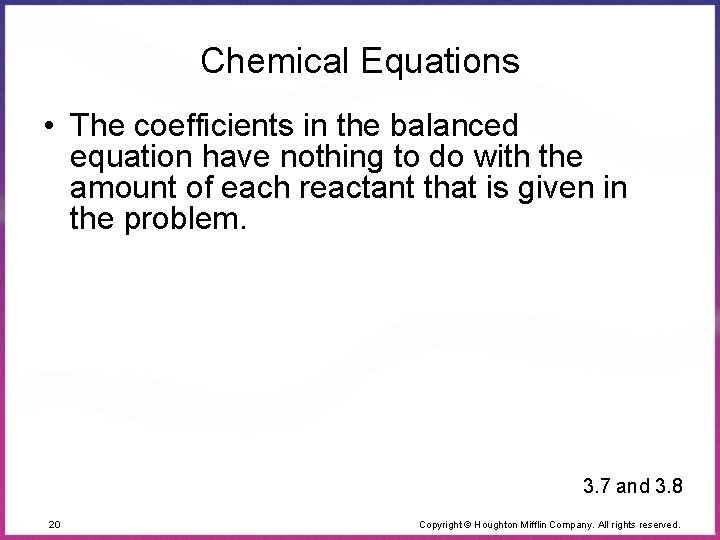Chemical Equations • The coefficients in the balanced equation have nothing to do with the amount of each reactant that is given in the problem. 3. 7 and 3. 8 20 Copyright © Houghton Mifflin Company. All rights reserved.Chemical Equations • The balanced equation represents a ratio of reactants and products, not what actually “happens” during a reaction. • Use the coefficients in the balanced equation to decide the amount of each reactant that is used, and the amount of each product that is formed. 3. 7 and 3. 8 21 Copyright © Houghton Mifflin Company. All rights reserved.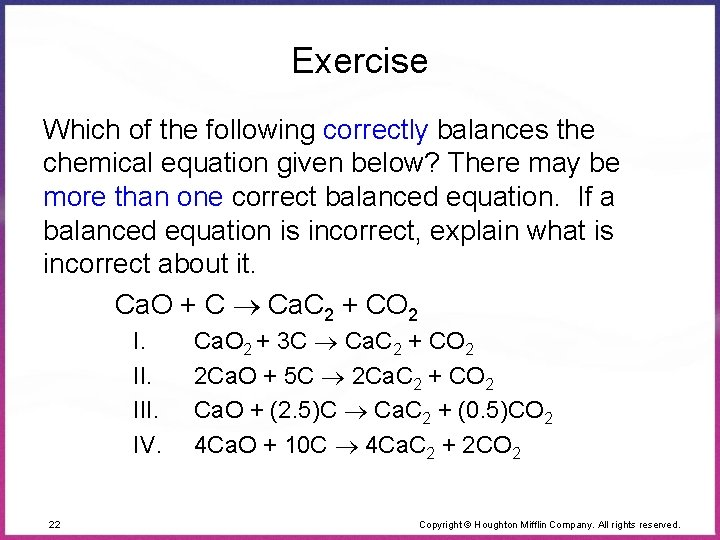Exercise Which of the following correctly balances the chemical equation given below? There may be more than one correct balanced equation. If a balanced equation is incorrect, explain what is incorrect about it. Ca. O + C Ca. C 2 + CO 2 I. II. IV. 22 Ca. O 2 + 3 C Ca. C 2 + CO 2 2 Ca. O + 5 C 2 Ca. C 2 + CO 2 Ca. O + (2. 5)C Ca. C 2 + (0. 5)CO 2 4 Ca. O + 10 C 4 Ca. C 2 + 2 CO 2 Copyright © Houghton Mifflin Company. All rights reserved.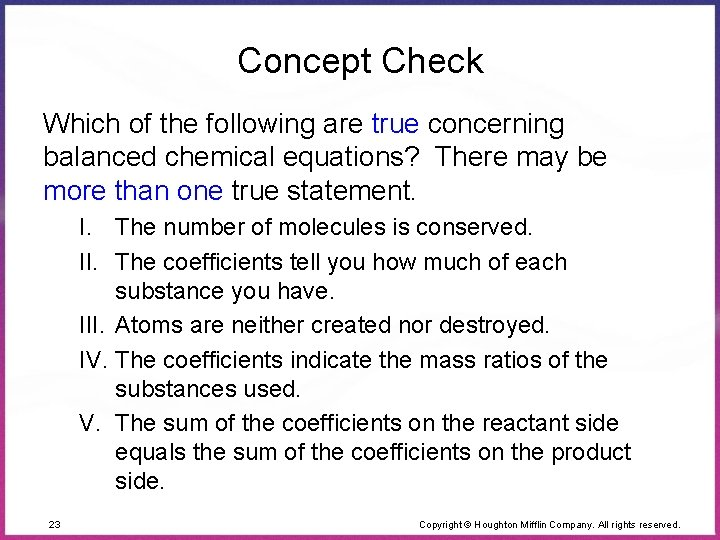Concept Check Which of the following are true concerning balanced chemical equations? There may be more than one true statement. I. The number of molecules is conserved. II. The coefficients tell you how much of each substance you have. III. Atoms are neither created nor destroyed. IV. The coefficients indicate the mass ratios of the substances used. V. The sum of the coefficients on the reactant side equals the sum of the coefficients on the product side. 23 Copyright © Houghton Mifflin Company. All rights reserved.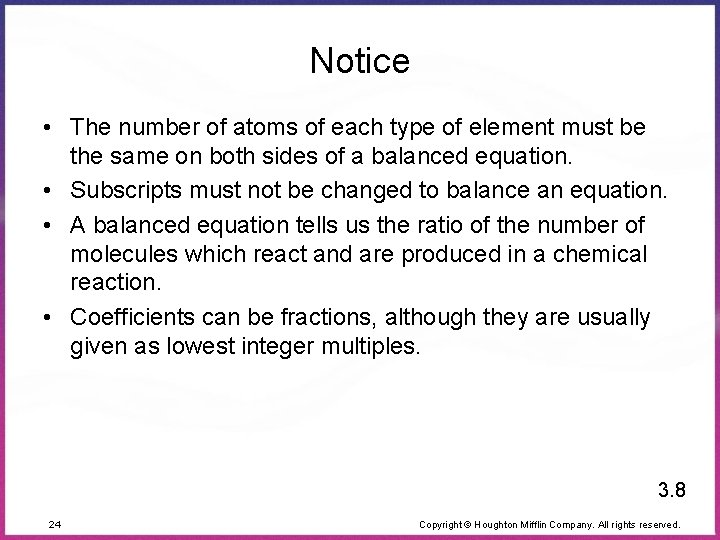Notice • The number of atoms of each type of element must be the same on both sides of a balanced equation. • Subscripts must not be changed to balance an equation. • A balanced equation tells us the ratio of the number of molecules which react and are produced in a chemical reaction. • Coefficients can be fractions, although they are usually given as lowest integer multiples. 3. 8 24 Copyright © Houghton Mifflin Company. All rights reserved.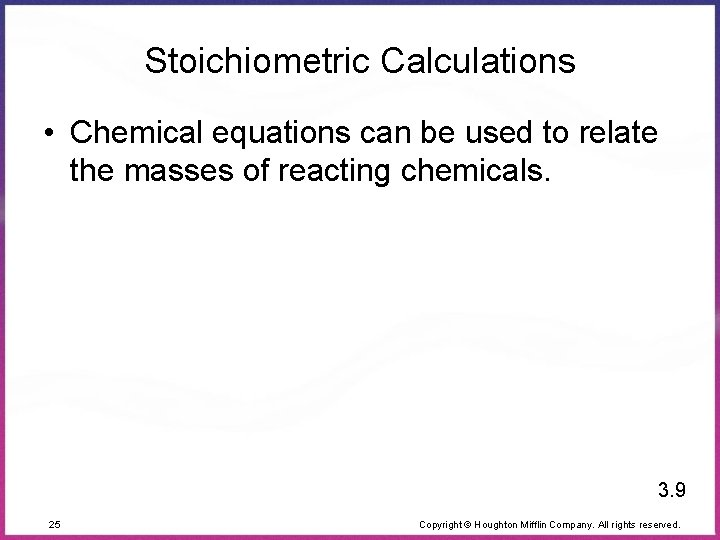Calculating Masses in Reactions • Balance the equation. • Convert mass to moles. • Set up mole ratios from the balanced equation. • Calculate number of moles of desired reactant or product. • Convert back to grams. 3. 9 26 Copyright © Houghton Mifflin Company. All rights reserved.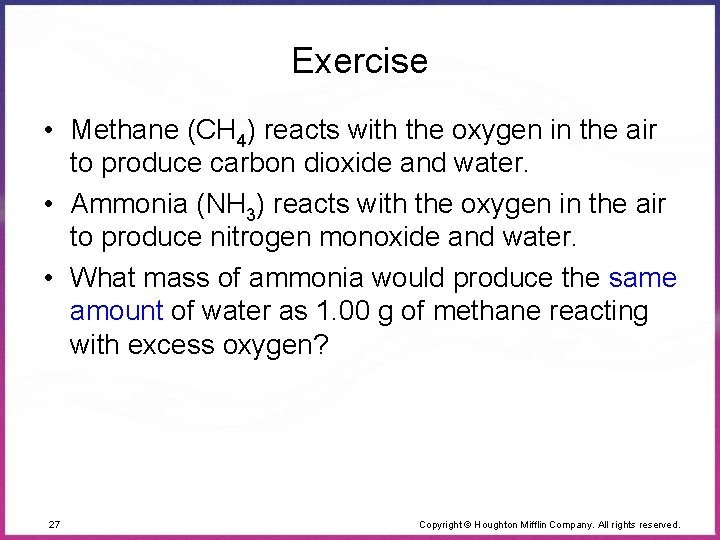Exercise • Methane (CH 4) reacts with the oxygen in the air to produce carbon dioxide and water. • Ammonia (NH 3) reacts with the oxygen in the air to produce nitrogen monoxide and water. • What mass of ammonia would produce the same amount of water as 1. 00 g of methane reacting with excess oxygen? 27 Copyright © Houghton Mifflin Company. All rights reserved.Let’s Think About It We need to know: – How much water is produced from 1. 00 g of methane and excess oxygen. – How much ammonia is needed to produce the amount of water calculated above. 28 Copyright © Houghton Mifflin Company. All rights reserved.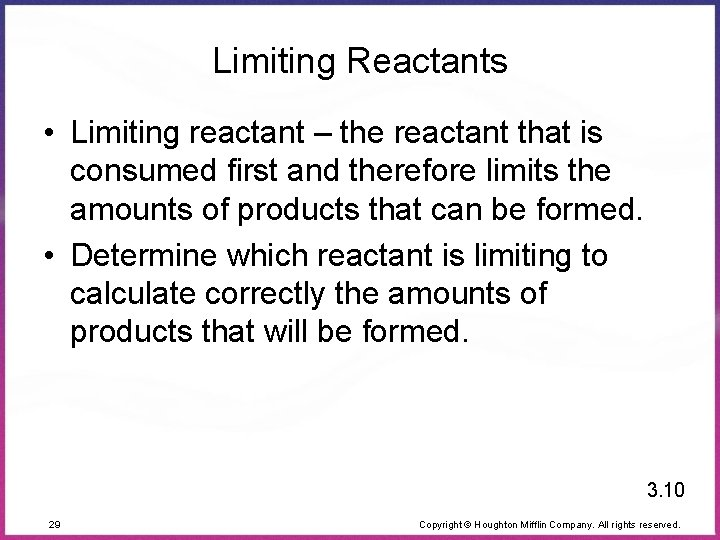Limiting Reactants • Limiting reactant – the reactant that is consumed first and therefore limits the amounts of products that can be formed. • Determine which reactant is limiting to calculate correctly the amounts of products that will be formed. 3. 10 29 Copyright © Houghton Mifflin Company. All rights reserved.Limiting Reactants • Methane and water will react to form products according to the equation: CH 4 + H 2 O 3 H 2 + CO 3. 10 31 Copyright © Houghton Mifflin Company. All rights reserved.Limiting Reactants • The amount of products that can form is limited by the methane. • Methane is the limiting reactant. • Water is in excess. 3. 10 34 Copyright © Houghton Mifflin Company. All rights reserved.Concept Check Which of the following reaction mixtures could produce the greatest amount of product? Each involves the reaction symbolized by the equation: 2 H 2 + O 2 2 H 2 O a) 2 moles of H 2 and 2 moles of O 2 b) 2 moles of H 2 and 3 moles of O 2 c) 2 moles of H 2 and 1 mole of O 2 d) 3 moles of H 2 and 1 mole of O 2 e) Each produce the same amount of product 35 Copyright © Houghton Mifflin Company. All rights reserved.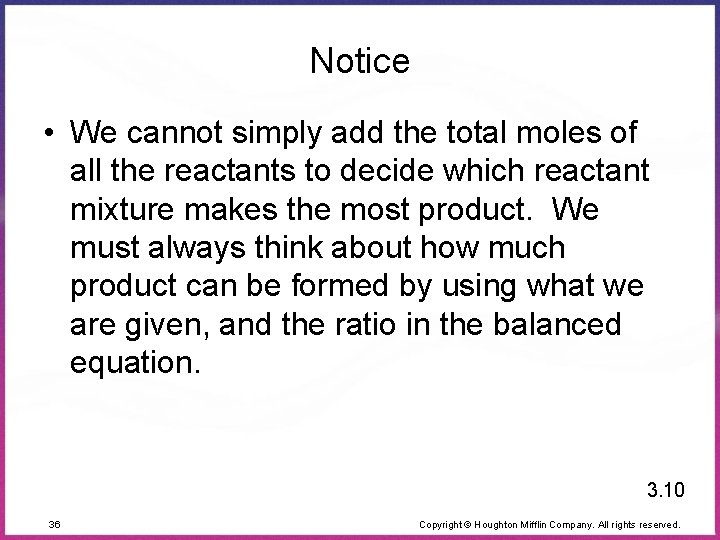Notice • We cannot simply add the total moles of all the reactants to decide which reactant mixture makes the most product. We must always think about how much product can be formed by using what we are given, and the ratio in the balanced equation. 3. 10 36 Copyright © Houghton Mifflin Company. All rights reserved.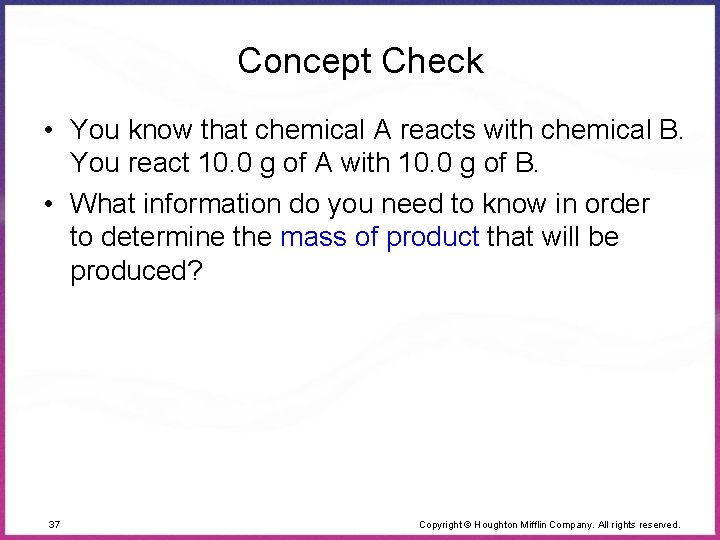Concept Check • You know that chemical A reacts with chemical B. You react 10. 0 g of A with 10. 0 g of B. • What information do you need to know in order to determine the mass of product that will be produced? 37 Copyright © Houghton Mifflin Company. All rights reserved.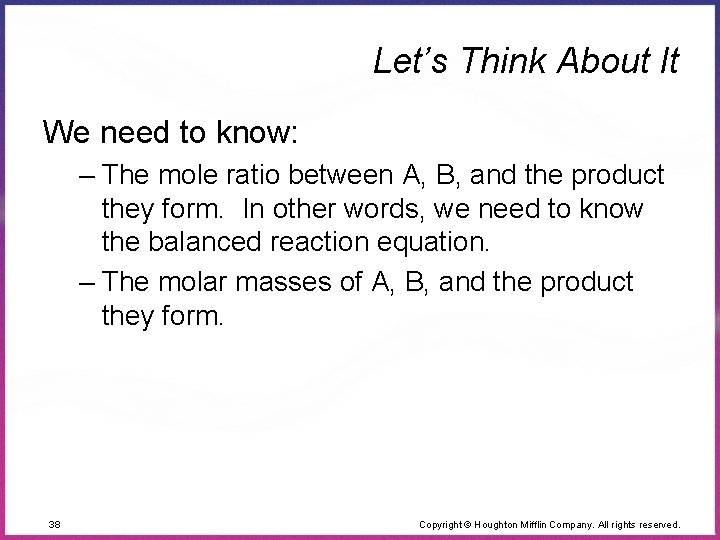Let’s Think About It We need to know: – The mole ratio between A, B, and the product they form. In other words, we need to know the balanced reaction equation. – The molar masses of A, B, and the product they form. 38 Copyright © Houghton Mifflin Company. All rights reserved.Exercise • You react 10. 0 g of A with 10. 0 g of B. What mass of product will be produced given that the molar mass of A is 10. 0 g/mol, B is 20. 0 g/mol, and C is 25. 0 g/mol? They react according to the equation: A + 3 B 2 C 39 Copyright © Houghton Mifflin Company. All rights reserved.